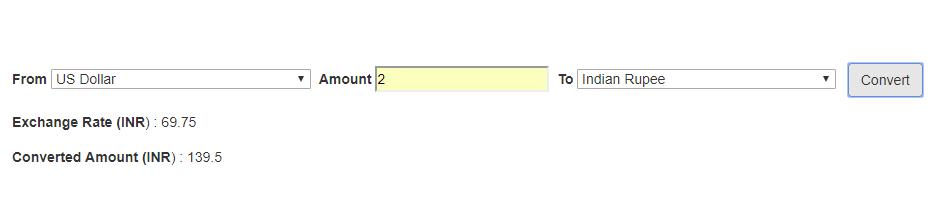# Build Currency Converter with PHP

Most of us use Google for searching web information. Previously Google provided currency converter API but now it is stopped. Now Google provides currency convert functionality through search to convert our desired currency to another. We can see the current status of our currency into other currency by going through Google's search currency converter page. However, sometimes we need to implement Google currency converter functionality into our application. This is very simple; you can implement it very easily with Google currency convert through search using PHP. So here in this tutorial, you will learn how to build currency converter in PHP using Google search.As we will cover this tutorial with live demo to build currency converter in PHP using Google search, so the file structure for this example is following.

• index.php

• ajax.js

• convert.php

• functions.php

Step1: Create Currency Convert Form
First in index.php file, we will create currency convert form to select from currency and to currency and input for amount to convert.

`<form method="post" id="currency-form"> <div class="form-group"> <label>From</label> <select name="from_currency"> <option value="INR">Indian Rupee</option> <option value="USD" selected="1">US Dollar</option> <option value="AUD">Australian Dollar</option> <option value="EUR">Euro</option> <option value="EGP">Egyptian Pound</option> <option value="CNY">Chinese Yuan</option> </select>  <label>Amount</label> <input type="text" placeholder="Currency" name="amount" id="amount" />  <label>To</label> <select name="to_currency"> <option value="INR" selected="1">Indian Rupee</option> <option value="USD">US Dollar</option> <option value="AUD">Australian Dollar</option> <option value="EUR">Euro</option> <option value="EGP">Egyptian Pound</option> <option value="CNY">Chinese Yuan</option> </select>   <button type="submit" name="convert" id="convert" class="btn btn-default">Convert</button> </div> </form> `

Step2: Handle Currency Convert Form Submit with Ajax
In ajax.js file, we will create a function handleCurrencyConvert() and call it on currency convert form submit to make Ajax request to convert.php to handle currency convert functionality at server end in PHP and display result using response.

`function handleCurrencyConvert() { var data = \$("#currency-form").serialize(); \$.ajax({ type : 'POST', url : 'convert.php', dataType:'json', data : data, beforeSend: function(){ \$("#convert").html('<span class="glyphicon glyphicon-transfer"></span>   converting ...'); }, success : function(response){ if(response.error == 1){ \$("#converted_rate").html('<span class="form-group has-error">Error: Please select different currency</span>'); \$("#converted_amount").html(""); \$("#convert").html('Convert'); \$("#converted_rate").show(); } else if(response.exhangeRate){ \$("#converted_rate").html("<strong>Exchange Rate ("+response.toCurrency+"</strong>) : "+response.exhangeRate); \$("#converted_rate").show(); \$("#converted_amount").html("<strong>Converted Amount ("+response.toCurrency+"</strong>) : "+response.convertedAmount); \$("#converted_amount").show(); \$("#convert").html('Convert'); } else { \$("#converted_rate").html("No Result"); \$("#converted_rate").show(); \$("#converted_amount").html(""); } } }); return false;}`

Step3: Handle Currency Conversion with PHP
In convert.php file, we will get currency form submit POST values and then call function currencyConverter() to convert currency. The function will return converted currency result in JSON format.
`<?phpif(isset(\$_POST['convert'])) { \$from_currency = trim(\$_POST['from_currency']); \$to_currency = trim(\$_POST['to_currency']); \$amount = trim(\$_POST['amount']); if(\$from_currency == \$to_currency) { \$data = array('error' => '1'); echo json_encode( \$data ); exit; } \$converted_currency=currencyConverter(\$from_currency, \$to_currency, \$amount); echo \$converted_currency;}?>`

Step4: Currency Convert PHP Function
In functions.php, we will create function currencyConverter(\$fromCurrency,\$toCurrency,\$amount) with three parameters. The first parameter is \$fromCurrency, the second is \$toCurrency means the currency in which it is converted. And third parameter is \$amount that will be passed for conversion. Here in this function, we will get the currency rate and then finally return total converted amount as JSON.

`<?phpfunction currencyConverter(\$fromCurrency,\$toCurrency,\$amount) { \$fromCurrency = urlencode(\$fromCurrency); \$toCurrency = urlencode(\$toCurrency); \$url = "https://www.google.com/search?q=".\$fromCurrency."+to+".\$toCurrency; \$get = file_get_contents(\$url); \$data = preg_split('/\D\s(.*?)\s=\s/',\$get); \$exhangeRate = (float) substr(\$data,0,7); \$convertedAmount = \$amount*\$exhangeRate; \$data = array( 'exhangeRate' => \$exhangeRate, 'convertedAmount' =>\$convertedAmount, 'fromCurrency' => strtoupper(\$fromCurrency), 'toCurrency' => strtoupper(\$toCurrency)); echo json_encode( \$data ); }?>`